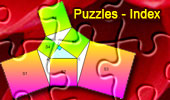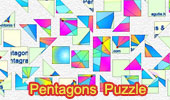Puzzles Online: Table of ContentPuzzle: Fields of Mathematics Mind Map. Clawson Point Puzzle, Geometry for Kids. 22 Piece Polygons Jigsaw Puzzle Ptolemy's Theorem. 22 Piece Polygons. Puzzle Geometry Problems 221 - 230. 22 Piece Polygons. Puzzle: Geometry Problems 161 - 170. 22 piece polygons. Area, Triangle, Parallelogram, Trapezoid, Pentagon, Diagonal, Midpoint, Octagon. Puzzle based on the Kosnita's Theorem Kosnita's Theorem. Circumcenters, Concurrent lines. Puzzle based on the problem 410: 22 piece polygons. Two Regular Pentagons, Angle. . Puzzle based on problem 401. Area, Triangle, Angle bisector, Circumcircle, Perpendicular bisector, Congruence. Puzzle based on the problem 368. Triangle, 120 degrees, Angle bisectors, Perpendicular. . Puzzle: Euclid's Elements Book I, 23 Definitions. One-page visual illustration. Puzzle: Inca Skull Surgery. 22 Piece Polygons. Inca Skull Surgeons Were "Highly Skilled," Study Finds. Trepanation = square hole in the skull. Jigsaw Puzzle: Pascal's Theorem 24 Pieces. The Road Not Taken Puzzle. Robert Frost's poem, puzzle 48 pieces of USA map. Puzzle: If - Rudyard Kipling' Poem. 6 piece classic. Puzzle: Marion Walter's theorem. Puzzle cut: 12 Piece ZigZag Based on Marion Walter's theorem Intouch and Extouch Triangles. Puzzle cut: 20 Piece Classic Based on Proposed Problem 86. Contact Triangles. Puzzle cut: 22 Piece Polygons Based on Proposed Problem 85.Pentagons & Pentagrams - Puzzle: 96 triangles. Puzzle of the Newton's Theorem: 50 pieces of circles. Euler's Formula - Puzzle: 55 pieces in a six star style of piece. Nazca Lines: The Monkey - Puzzle. Nazca Lines: The Condor bird. Puzzle: 40 pieces of birds tessellation. Nazca Lines: The Spider. Puzzle: 44 pieces in a tri-dove style of piece. Puzzle of the Nagel Point: 22 pieces of polygons. Equal Incircles Theorem: 20 Piece Classic. Puzzle of the Ceva's Theorem: 48 classic piece. Morley's Theorem Puzzle: 22 pieces of polygons. The Lord of Sipan, Spiderman Puzzle: 50 pieces of circles. The Lord of Sipan Puzzle: 22 pieces of polygons. An example of Inca skill with polygonal masonry. Puzzle: 22 pieces of polygons. Machu Picchu. Lost City of the Incas. Puzzle: Puzzle 41 pieces of polyominoes. Jigsaw Puzzle: Problem 1182: Two Squares. 35 Pieces.